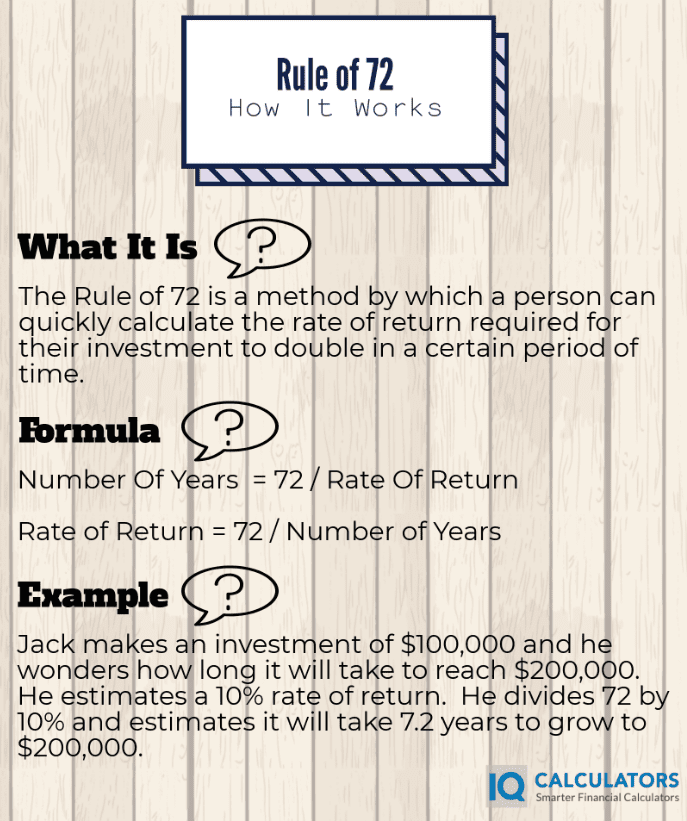# What Is The Rule of 72

The rule of 72 is a formula that investors and financial analysts sometimes use in order to quickly calculate how quickly their money will double in value.  Conversely, the rule of 72 formula can also be used to calculate what rate of return is required to double your money in a defined period of time.  The rule of 72 is a valuable tool for the most experienced and novice investors alike.  You shouldn't leave home without it.

## Rule of 72 Formula

When solving for number of years = 72 divided by Rate of Return

When solving for rate of return = 72 divided by Number of Years

### Example When Solving For Years

Susan wants to invest \$100,000 in a new project.  She believes she will get an annual rate of return of 10 percent.  She wants to know how long it will take for her initial investment to double.  So she applies the rule of 72 by dividing 72 by 10.  Quickly she solves that it will take 7.2 years for her investment to double.

### Example When Solving For Rate of Return

Eric wants to invest \$100,000 dollars in a new project.  Eric knows that according to his metrics, any good investment should double in at least 10 years.  He wants to know what rate of return he will need to double his investment in that amount of time.  By using the rule of 72, he divides 72 by 10 and gets 7.2.  Quickly he solves that in order for his investment to double in 10 years, he will need at least a 7.2% rate of return.

## Difference Between Rule of 70 and 72

The rule of 70 and rule of 72 are the same thing.  Some people prefer to use 70 over the number 72 because it is slightly more accurate but the rule of 72 seems to be more popular probably because it has a better ring to it.  In order to adjust for even greater accuracy, use the rule of 69.3.  The rule of 69.3 will get you to almost the exact number of years or rate of return depending on which one you are solving for.  Its not as convenient to use a number with decimals when trying to solve a simple equation though.  If you want to make things the simplest, use our rule of 72 calculator.## Conclusion

If you need a quick and easy way to calculate the rate of return needed to double your money, or the amount of time needed to double your money, then the rule of 72 is the calculation you're looking for.   The rule of 72 isn't the most accurate number you could use.  In fact, 69.3 is the most accurate, but the rule of 72 keeps things simple.

The rule of 72 is as simple as a finance calculation there is, but if you are looking to do more in depth financial analysis, you may want to look at using the payback period, net present value, or the internal rate of return calculator to do your analysis.  We hope you find this article helpful and be sure to leave a comment in the comment section.